Next: Clausius-Mossotti Relation Up: Electrostatics in Dielectric Media Previous: Energy Density Within Dielectric

# Force Density Within Dielectric Medium

Equation (567) was derived by considering a virtual process in which true charges are added to a system of charges and dielectrics that are held fixed, so that no mechanical work is done against physical displacements. Consider a different virtual process in which the physical coordinates of the charges and dielectric are given a virtual displacement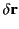at each point in space, but no free charges are added to the system. Because we are dealing with a conservative system, the energy expression (567) can still be employed, despite the fact that it was derived in terms of another virtual process. The variation in the total electrostatic energy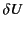when the system undergoes a virtual displacementis related to the electrostatic force density,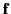, acting within the dielectric medium via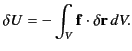(568)

If the medium is moving with a velocity field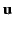then the rate at which electrostatic energy is drained from the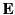and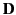fields is given by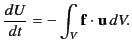(569)

Consider the energy increment due to a change,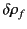, in the free charge distribution, and a change,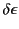, in the dielectric constant, which are both assumed to be caused by the virtual displacement. From Equation (567),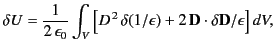(570)

or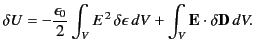(571)

Here, the first term represents the energy increment due to the change in dielectric constant associated with the virtual displacement, whereas the second term corresponds to the energy increment caused by displacement of the free charges. The second term can be written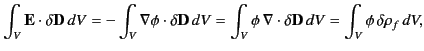(572)

where surface terms have been neglected. Thus, Equation (572) implies that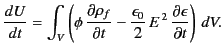(573)

In order to arrive at an expression for the force density,, we need to express the time derivatives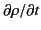and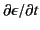in terms of the velocity field,. This can be achieved by adopting a dielectric equation of state: that is, a relation that specifies the dependence of the dielectric constant,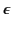, on the mass density,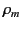. Let us assume that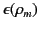is a known function. It follows that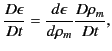(574)

where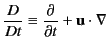(575)

is the total time derivative (i.e., the time derivative in a frame of reference that is locally co-moving with the dielectric.) The hydrodynamic equation of continuity of the dielectric is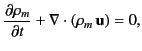(576)

which implies that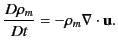(577)

It follows that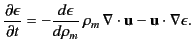(578)

The conservation equation for the free charges is written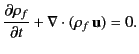(579)

Thus, we can express Equation (574) in the form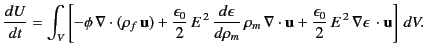(580)

Integrating the first term by parts, and neglecting any surface contributions, we obtain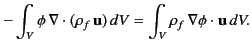(581)

Likewise,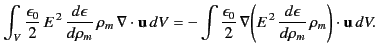(582)

Thus, Equation (581) becomes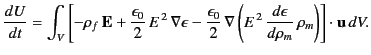(583)

Comparing with Equation (570), we deduce that the force density inside the dielectric is given by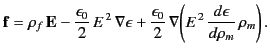(584)

The first term in the above equation is the standard electrostatic force density (due to the presence of free charges). The second term represents a force that appears whenever an inhomogeneous dielectric is placed in an electric field. The last term, which is known as the electrostriction term, gives a force acting on a dielectric in an inhomogeneous electric field. Note that the magnitude of the electrostriction force density depends explicitly on the dielectric equation of state of the material, through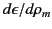. The electrostriction term gives zero net force acting on any finite region of dielectric, provided we can integrate over a large enough portion of the dielectric that its extremities lie in a field-free region. For this reason, the term is frequently omitted, because in the calculation of the total forces acting on dielectric bodies it usually makes no contribution. Note, however, that if the electrostriction term is omitted then an incorrect pressure variation within the dielectric is obtained, even though the total force is given correctly.Next: Clausius-Mossotti Relation Up: Electrostatics in Dielectric Media Previous: Energy Density Within Dielectric
Richard Fitzpatrick 2014-06-27